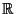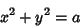## Hasse Principle

A collection of equations satisfies the Hasse principle if, whenever one of the equations has solutions inand all the, then the equations have solutions in the Rationals. Examples include the set of equationswith,, andIntegers, and the set of equationsforrational. The trivial solutionis usually not taken into account when deciding if a collection of homogeneous equations satisfies the Hasse principle. The Hasse principle is sometimes called the Local-Global Principle.